Like   Tweet   in

# President's Note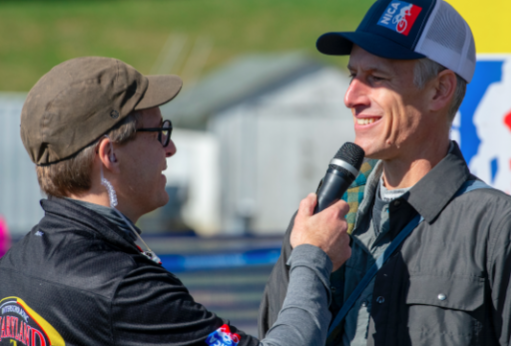Catching up with Maryland announcer, Ray at the Maryland League's Championship event. Photo: Antonio D Paterniti

The just completed fall season has been our biggest yet for NICA, we had over 13,000 student-athletes racing across fifteen states, which included three new leagues. I had the opportunity to attend the Maryland League’s Championship event and was impressed by the team spirit exhibited by the student-athletes on the race course and in the pit zone, as well as the professionalism of the race crew. November is a month for giving thanks, and, on behalf of the NICA National Staff and League Directors, I want to express how extremely thankful we all are to be part of a nationwide cycling community that is inspiring a future generation of cyclists while also positively changing lives through cycling.

Seeing the images and hearing stories from participants across NICA’s fall leagues emphasizes that NICA’s weekend competitions are the culmination of many hours spent doing what we love: riding bikes with our friends. We know that getting middle and high school students on bikes not only increases the likelihood that they will continue riding into adulthood, but also boosts bicycling among friends and family members too.

In recognition of this fact, People For Bikes has been a supporter of NICA’s mission and programs. Our shared commitment is to make bike riding safer and easier – on mountain bike trails and on city streets (and everything in between), for weekend races and to work and school. People For Bikes accomplishes this core mission by working with community residents, city leaders, elected officials and national partners like NICA. This season, we ask that you take a moment and add your voice to the 1.3 million PeopleForBikes members who support better biking where they live. (It’s easy and free.) When people ride bikes, great things happen.

Sincerely,
Austin & Tim Blumenthal, President, People for Bikes

 table div table+table+table+table div table{width:100%;padding:0}table div table+table+table+table div table img{width:96.23%;padding:0;float:none}table div table+table+table+table div table td{width:100%;padding:0 1.88% 18px}/* styles */# What's Happening Across America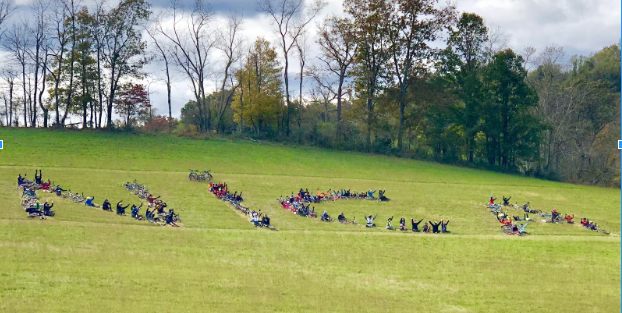West Virginia Student-Athletes at their final race of the season. Photo: Kyle Mandler

## Fall 2018 Race Season a Huge Success!

Congratulations to the 13,626 student-athletes, 6,485 coaches and countless volunteers who made our fall race season a success. From August through November, there were 76 events across 15 states!

We'd like to give a congratulations to Oregon, West Virginia and Maryland for phenomenally successful inaugural seasons. We caught up with their League Directors to get a take on their first seasons with NICA HERE.

 table div table+table+table+table+table+table+table div table{width:100%;padding:0}table div table+table+table+table+table+table+table div table img{width:96.23%;padding:0;float:none}table div table+table+table+table+table+table+table div table td{width:100%;padding:0 1.88% 18px}/* styles */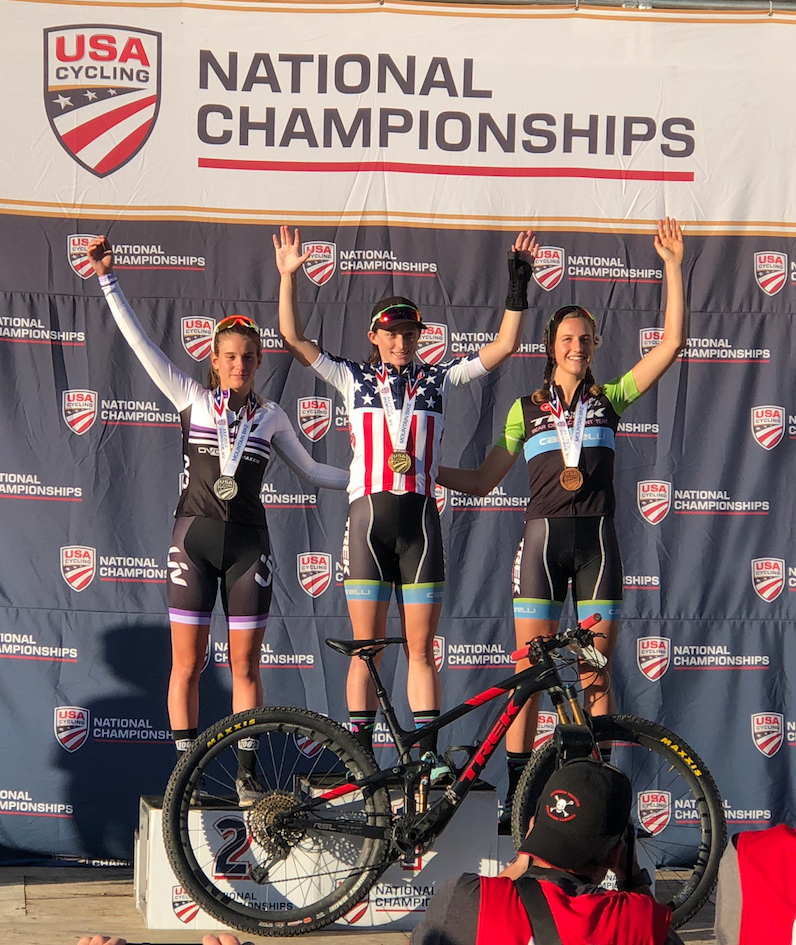# Development Teams provide elite competition opportunities for NICA student-athletes

NICA is changing the shape of youth cycling across the United States, and elite development programs like the Bear Development Team, Durango Devo, and others are working with NICA student-athletes to help them compete at the elite level both nationally and internationally. Learn more about these opportunities for NICA student-athletes HERE.

 table div table+table+table+table+table+table+table+table+table div table{width:100%;padding:0}table div table+table+table+table+table+table+table+table+table div table img{width:96.23%;padding:0;float:none}table div table+table+table+table+table+table+table+table+table div table td{width:100%;padding:0 1.88% 18px}/* styles */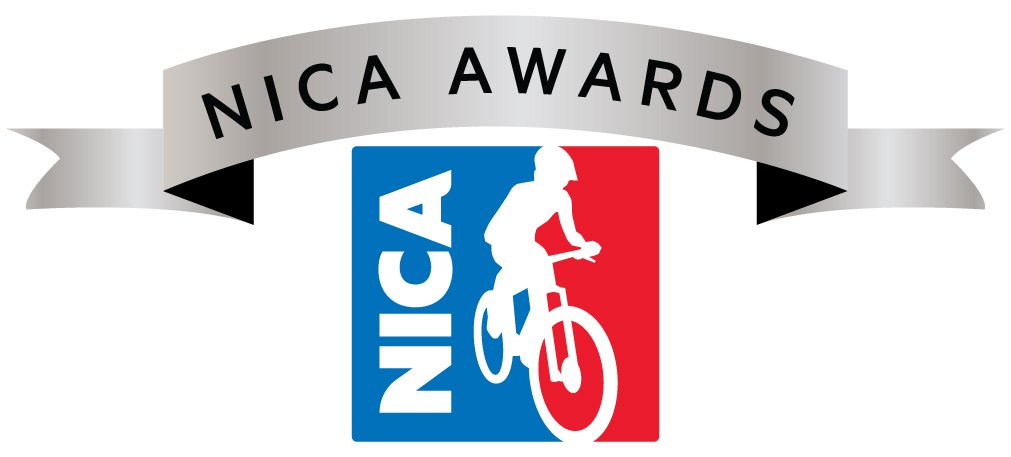# 2018 NICA Award Nominations Open

Each year we recognize student-athletes, coaches, volunteers, individuals and partners that have made outstanding contributions to the youth mountain biking movement across the country. Awards are given out for contributions to the NICA community, outstanding coaching, student-athlete leadership, athleticism and for those showing courage and contributing to NICA's legacy. Nominations will be accepted through 12/31/2018. For more information on the individual awards and on the nomination submission process, please click HERE.

 table div table+table+table+table+table+table+table+table+table+table+table div table{width:100%;padding:0}table div table+table+table+table+table+table+table+table+table+table+table div table img{width:96.23%;padding:0;float:none}table div table+table+table+table+table+table+table+table+table+table+table div table td{width:100%;padding:0 1.88% 18px}/* styles */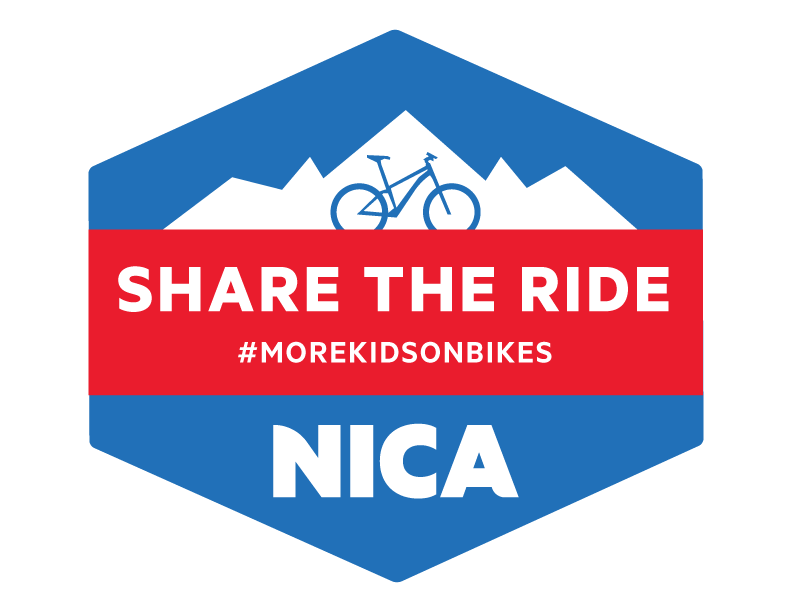# Help us Share the Ride this Holiday Season starting on Giving Tuesday!

This Nov 27th is #GivingTuesday. We are fundraising to support our mission of building strong minds, bodies, character, and communities through cycling. Mark your calendar and help us reach our fundraising goals to get #morekidsonbikes!

 table div table+table+table+table+table+table+table+table+table+table+table+table+table div table{width:100%;padding:0}table div table+table+table+table+table+table+table+table+table+table+table+table+table div table img{width:96.23%;padding:0;float:none}table div table+table+table+table+table+table+table+table+table+table+table+table+table div table td{width:100%;padding:0 1.88% 18px}/* styles */# Support NICA with AmazonSmile

Did you know that when you register with AmazonSmile and select NICA as your nonprofit, every time you make an eligible purchase, Amazon donates 0.5% of your purchase price to NICA? Shop at smile.amazon.com and Amazon will donate to NICA! Learn more or sign up HERE.

 table div table+table+table+table+table+table+table+table+table+table+table+table+table+table+table div table{width:100%;padding:0}table div table+table+table+table+table+table+table+table+table+table+table+table+table+table+table div table img{width:96.23%;padding:0;float:none}table div table+table+table+table+table+table+table+table+table+table+table+table+table+table+table div table td{width:100%;padding:0 1.88% 18px}/* styles */# Trek Student-Athlete Purchase Program

NICA student-athletes are now eligible for great discounts on select Trek mountain bikes and Bontrager gear from their local Trek retailer. Click HERE to find your local participating Trek retailer and learn about the sweet deals for registered NICA athletes and coaches.

 table.module-16{width:75.47%;padding:0}table div table+table+table+table+table+table+table+table+table+table+table+table+table+table+table+table+table div table{width:75.47%;float:none;margin-left:auto;margin-right:auto;padding:0}table div table+table+table+table+table+table+table+table+table+table+table+table+table+table+table+table+table div table a{border:0 none;text-decoration:none}table div table+table+table+table+table+table+table+table+table+table+table+table+table+table+table+table+table div table img{width:100%!important;border:0 none;text-decoration:none}table div table+table+table+table+table+table+table+table+table+table+table+table+table+table+table+table+table div table td{width:100%;padding:0}/* styles */
 table div table+table+table+table+table+table+table+table+table+table+table+table+table+table+table+table+table+table div table{width:100%;padding:0}table div table+table+table+table+table+table+table+table+table+table+table+table+table+table+table+table+table+table div table img{width:96.23%;padding:0;float:none}table div table+table+table+table+table+table+table+table+table+table+table+table+table+table+table+table+table+table div table td{width:100%;padding:0 1.88% 18px}/* styles */## Stay Safe with RoadID

ROAD iD, believes in making a positive difference in the world. They mainly do this by creating awesome products that save lives and empower adventure, but they also love supporting causes that they believe in and that share their mission.

RoadID is excited to partner with NICA and is offering NICA student-athletes a 35% discount on their selection of ID bands and accessories. Sign up HERE to get your discount!

 table div table+table+table+table+table+table+table+table+table+table+table+table+table+table+table+table+table+table+table+table div table{width:100%;padding:0}table div table+table+table+table+table+table+table+table+table+table+table+table+table+table+table+table+table+table+table+table div table img{width:96.23%;padding:0;float:none}table div table+table+table+table+table+table+table+table+table+table+table+table+table+table+table+table+table+table+table+table div table td{width:100%;padding:0 1.88% 18px}/* styles */## NICA Welcomes New Corporate Boosters

We're excited to welcome GUP, and Kenda Tires to NICA Corporate Booster program. Our corporate boosters support NICA's mission through donations, in-kind gifts and proceeds of product sales. Learn more about our Corporate Booster Club HERE.

 table div table+table+table+table+table+table+table+table+table+table+table+table+table+table+table+table+table+table+table+table+table+table div table{width:100%;padding:0}table div table+table+table+table+table+table+table+table+table+table+table+table+table+table+table+table+table+table+table+table+table+table div table img{width:96.23%;padding:0;float:none}table div table+table+table+table+table+table+table+table+table+table+table+table+table+table+table+table+table+table+table+table+table+table div table td{width:100%;padding:0 1.88% 18px}/* styles */# Interested in Partnering with NICA in 2019?

When you become a NICA Partner, you help to foster the development of NICA and support the expansion of new mountain biking leagues across the country. Your commitment to NICA has a huge impact on our student-athletes, their families and communities across the nation. We are currently looking for Corporate Sponsors and Boosters for the 2019 season. Please contact Kristine@nationalmtb.org or reply to this newsletter to discuss more.

 table div table+table+table+table+table+table+table+table+table+table+table+table+table+table+table+table+table+table+table+table+table+table+table+table div table{width:100%;padding:0}table div table+table+table+table+table+table+table+table+table+table+table+table+table+table+table+table+table+table+table+table+table+table+table+table div table img{width:96.23%;padding:0;float:none}table div table+table+table+table+table+table+table+table+table+table+table+table+table+table+table+table+table+table+table+table+table+table+table+table div table td{width:100%;padding:0 1.88% 18px}/* styles */# NICA in Your Neighborhood

## We LOVE seeing what our student-athletes, teams, coaches and leagues are up to across the country. Here are some of the highlights from the past month!

Do you have a great photo worthy of inclusion in a future issue of NICA News? If so, please email to media@nationalmtb.org and you may see it published! And don't forget - when you're posting your photos to tag @nationalmtb #morekidsonbikes for a chance to see your photo in NICA News!

 table.module-25{width:75.85%;padding:0}table div table+table+table+table+table+table+table+table+table+table+table+table+table+table+table+table+table+table+table+table+table+table+table+table+table+table div table{width:75.85%;float:none;margin-left:auto;margin-right:auto;padding:0}table div table+table+table+table+table+table+table+table+table+table+table+table+table+table+table+table+table+table+table+table+table+table+table+table+table+table div table a{border:0 none;text-decoration:none}table div table+table+table+table+table+table+table+table+table+table+table+table+table+table+table+table+table+table+table+table+table+table+table+table+table+table div table img{width:100%!important;border:0 none;text-decoration:none}table div table+table+table+table+table+table+table+table+table+table+table+table+table+table+table+table+table+table+table+table+table+table+table+table+table+table div table td{width:100%;padding:0}/* styles */
 /* styles */ For @yrayelizabeth93 there was no better way to wrap up the season than with a sprint finish and an amazing team to cheer on during @minnesotahighschoolmtb state championship weekend!
 table.module-27{width:75.47%;padding:0}table div table+table+table+table+table+table+table+table+table+table+table+table+table+table+table+table+table+table+table+table+table+table+table+table+table+table+table+table div table{width:75.47%;float:none;margin-left:auto;margin-right:auto;padding:0}table div table+table+table+table+table+table+table+table+table+table+table+table+table+table+table+table+table+table+table+table+table+table+table+table+table+table+table+table div table a{border:0 none;text-decoration:none}table div table+table+table+table+table+table+table+table+table+table+table+table+table+table+table+table+table+table+table+table+table+table+table+table+table+table+table+table div table img{width:100%!important;border:0 none;text-decoration:none}table div table+table+table+table+table+table+table+table+table+table+table+table+table+table+table+table+table+table+table+table+table+table+table+table+table+table+table+table div table td{width:100%;padding:0}/* styles */
 /* styles */ @bryan_pendergraftmtb - "What an amazing last race for my NICA career. I can not say how much fun I have had with my team and fellow racers and I am so thankful for the friendships I’ve made"
 table.module-29{width:75.47%;padding:0}table div table+table+table+table+table+table+table+table+table+table+table+table+table+table+table+table+table+table+table+table+table+table+table+table+table+table+table+table+table+table div table{width:75.47%;float:none;margin-left:auto;margin-right:auto;padding:0}table div table+table+table+table+table+table+table+table+table+table+table+table+table+table+table+table+table+table+table+table+table+table+table+table+table+table+table+table+table+table div table a{border:0 none;text-decoration:none}table div table+table+table+table+table+table+table+table+table+table+table+table+table+table+table+table+table+table+table+table+table+table+table+table+table+table+table+table+table+table div table img{width:100%!important;border:0 none;text-decoration:none}table div table+table+table+table+table+table+table+table+table+table+table+table+table+table+table+table+table+table+table+table+table+table+table+table+table+table+table+table+table+table div table td{width:100%;padding:0}/* styles */
 /* styles */ Utah Varsity Girls cranking out watts at the top of the climb during state championships in St. George - @racelicious_mtb is a fan of the action!
 table.module-31{width:75.66%;padding:0}table div table+table+table+table+table+table+table+table+table+table+table+table+table+table+table+table+table+table+table+table+table+table+table+table+table+table+table+table+table+table+table+table div table{width:75.66%;float:none;margin-left:auto;margin-right:auto;padding:0}table div table+table+table+table+table+table+table+table+table+table+table+table+table+table+table+table+table+table+table+table+table+table+table+table+table+table+table+table+table+table+table+table div table a{border:0 none;text-decoration:none}table div table+table+table+table+table+table+table+table+table+table+table+table+table+table+table+table+table+table+table+table+table+table+table+table+table+table+table+table+table+table+table+table div table img{width:100%!important;border:0 none;text-decoration:none}table div table+table+table+table+table+table+table+table+table+table+table+table+table+table+table+table+table+table+table+table+table+table+table+table+table+table+table+table+table+table+table+table div table td{width:100%;padding:0}/* styles */
 /* styles */ @mikefrankgarry has loved working with his @wisconsinmtb crew of athletes and coaches (and dinosaurs). Photo: @brettstepanik
 table.module-33{width:75.47%;padding:0}table div table+table+table+table+table+table+table+table+table+table+table+table+table+table+table+table+table+table+table+table+table+table+table+table+table+table+table+table+table+table+table+table+table+table div table{width:75.47%;float:none;margin-left:auto;margin-right:auto;padding:0}table div table+table+table+table+table+table+table+table+table+table+table+table+table+table+table+table+table+table+table+table+table+table+table+table+table+table+table+table+table+table+table+table+table+table div table a{border:0 none;text-decoration:none}table div table+table+table+table+table+table+table+table+table+table+table+table+table+table+table+table+table+table+table+table+table+table+table+table+table+table+table+table+table+table+table+table+table+table div table img{width:100%!important;border:0 none;text-decoration:none}table div table+table+table+table+table+table+table+table+table+table+table+table+table+table+table+table+table+table+table+table+table+table+table+table+table+table+table+table+table+table+table+table+table+table div table td{width:100%;padding:0}/* styles */
 /* styles */ How do you get #moregirlsonbikes? According to Coach @beckybatizy you don’t create a team, you build a community. It starts with a whisper and ends with laughter. Photo: @lindaguerrettephotography
 table.module-35{width:75.28%;padding:0}table div table+table+table+table+table+table+table+table+table+table+table+table+table+table+table+table+table+table+table+table+table+table+table+table+table+table+table+table+table+table+table+table+table+table+table+table div table{width:75.28%;float:none;margin-left:auto;margin-right:auto;padding:0}table div table+table+table+table+table+table+table+table+table+table+table+table+table+table+table+table+table+table+table+table+table+table+table+table+table+table+table+table+table+table+table+table+table+table+table+table div table a{border:0 none;text-decoration:none}table div table+table+table+table+table+table+table+table+table+table+table+table+table+table+table+table+table+table+table+table+table+table+table+table+table+table+table+table+table+table+table+table+table+table+table+table div table img{width:100%!important;border:0 none;text-decoration:none}table div table+table+table+table+table+table+table+table+table+table+table+table+table+table+table+table+table+table+table+table+table+table+table+table+table+table+table+table+table+table+table+table+table+table+table+table div table td{width:100%;padding:0}/* styles */
 /* styles */ The anticipation builds for @cheeriosmtb and @hals_und_bikes as they wait to start a half-time race at their local high school football game!
 table div table+table+table+table+table+table+table+table+table+table+table+table+table+table+table+table+table+table+table+table+table+table+table+table+table+table+table+table+table+table+table+table+table+table+table+table+table+table div table{width:100%;padding:0}table div table+table+table+table+table+table+table+table+table+table+table+table+table+table+table+table+table+table+table+table+table+table+table+table+table+table+table+table+table+table+table+table+table+table+table+table+table+table div table img{width:96.23%;padding:0;float:none}table div table+table+table+table+table+table+table+table+table+table+table+table+table+table+table+table+table+table+table+table+table+table+table+table+table+table+table+table+table+table+table+table+table+table+table+table+table+table div table td{width:100%;padding:0 1.88% 18px}/* styles */table div table+table+table+table+table+table+table+table+table+table+table+table+table+table+table+table+table+table+table+table+table+table+table+table+table+table+table+table+table+table+table+table+table+table+table+table+table+table+table div table td,table.module-38{width:100%;padding:0}table div table+table+table+table+table+table+table+table+table+table+table+table+table+table+table+table+table+table+table+table+table+table+table+table+table+table+table+table+table+table+table+table+table+table+table+table+table+table+table div table{width:100%;float:none;margin-left:auto;margin-right:auto;padding:0}table div table+table+table+table+table+table+table+table+table+table+table+table+table+table+table+table+table+table+table+table+table+table+table+table+table+table+table+table+table+table+table+table+table+table+table+table+table+table+table div table a{border:0 none;text-decoration:none}table div table+table+table+table+table+table+table+table+table+table+table+table+table+table+table+table+table+table+table+table+table+table+table+table+table+table+table+table+table+table+table+table+table+table+table+table+table+table+table div table img{width:100%!important;border:0 none;text-decoration:none}/* styles */